# The comma is frequently used in C as a simple punctuation mark, to separate variable declarations, function arguments, etc. In certain situations, the comma acts as an operator.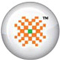PRO DATA DOCTOR it | es | pt | fr | de | jp | kr | cn | ru | nl | gr

The Comma Operator

The comma is frequently used in C as a simple punctuation mark, to separate variable declarations, function arguments, etc. In certain situations, the comma acts as an operator.

You can form an expression by separating two sub expressions with a comma. The result is as follows:

• Both expressions are evaluated, with the left expression being evaluated first.
• The entire expression evaluates to the value of the right expression.
For example, the following statement assigns the value of b to x, then increments a, and then increments b:
x = (a++, b++);

C operator precedence (Summary of C operators)

 Rank and Associativity Operators 1(left to right) () [] -> . 2(right to left) ! ~ ++ -- * (indirection) & (address-of) (type) sizeof + (unary) - (unary) 3(left to right) * (multiplication) / % 4(left to right) + - 5(left to right) << >> 6(left to right) < <= > >= 7(left to right) = = != 8(left to right) & (bitwise AND) 9(left to right) ^ 10(left to right) | 11(left to right) && 12(left to right) || 13(right to left) ?: 14(right to left) = += -= *= /= %= &= ^= |= <<= >>= 15(left to right) , () is the function operator; [] is the array operator.

Let us take an example of use of operators:

/* Use Of Operators */

int main()
{
int x = 0, y = 2, z = 1025;
float a = 0.0, b = 3.14159, c = -37.234;

/* incrementing */

x = x + 1; /* This increments x */
x++; /* This increments x */
++x; /* This increments x */
z = y++; /* z = 2, y = 3 */
z = ++y; /* z = 4, y = 4 */

/* decrementing */

y = y - 1; /* This decrements y */
y--; /* This decrements y */
--y; /* This decrements y */
y = 3;
z = y--; /* z = 3, y = 2 */
z = --y; /* z = 1, y = 1 */

/* arithmetic op */

a = a + 12; /* This adds 12 to a */
a += 12; /* This adds 12 more to a */
a *= 3.2; /* This multiplies a by 3.2 */
a -= b; /* This subtracts b from a */
a /= 10.0; /* This divides a by 10.0 */

/* conditional expression */

a = (b >= 3.0 ? 2.0 : 10.5 ); /* This expression */

if (b >= 3.0) /* And this expression */
a = 2.0; /* are identical, both */

else /* will cause the same */
a = 10.5; /* result. */

c = (a > b ? a : b); /* c will have the max of a or b */
c = (a > b ? b : a); /* c will have the min of a or b */

printf("x=%d, y=%d, z= %d\n", x, y, z);
printf("a=%f, b=%f, c= %f", a, b, c);

return 0;
}

and the result of this program will be displayed on the screen as:

 x=3, y=1, z=1 a=2.000000, b=3.141590, c=2.000000

Sample Chapters from book DATA RECOVERY WITH AND WITHOUT PROGRAMMING by Author Tarun Tyagi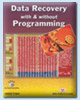Publishers of the Book Number of Pages ISBN Price of the Book BPB Publications, New Delhi, India 540 81-7656-922-4 \$69.00 (Including Shipping Charges, Cost of Book and Other expenses, Free Source Code CD included with the Book)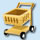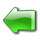page 1 | 2 | 3 | 4 | 5 | 6 | 7 | 8 | 9 | 10 | 11 | 12 | 13 | 14 | 15 | 16 | 17 | 18 | 19 | 20

 page 21 | 22 | 23 | 24 | 25 | 26 | 27 | 28 | 29 | 30 | 31 | 32 | 33 | 34 | 35 | 36 | 37

 page 38 | 39 | 40 | 41 | 42 | 43 | 44 | 45 | 46 | 47 | 48 | 49 | 50 | 51 | 52 | 53 | 54

 page 55 | 56 | 57 | 58 | 59 | 60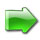© Copyright 2002-2005 DataDoctor.Biz
 Website Data Recovery | recuperación de Datos | Récupération de données | Datenrettung | Recupero dati | データ復旧 | 데이터 복구 | 数据恢复 | Восстановление данных | De terugwinning van gegevens | Ανάκτηση δεδομένων Sitemap Site map1 2 3 4 | Spanish1 2 3 | French1 2 3 | German1 2 3 | Italian1 2 3 | Portuguese1 2 3 | Japanese1 2 3 | Korean1 2 3 | Chinese1 2 3 | Russian1 2 3 | Dutch1 2 3 | Greek1 2 3 Data Recovery Book English | Spanish | French | German | Italian | Portuguese | Japanese | Korean | Chinese | Russian | Dutch | Greek